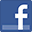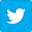Question ID: 1864 8/11 PREVIOUS QUESTION NEXT QUESTION Apply the following number format on Quantities: if the entry is zero, the respective cell will display the word Zero. If the entry is greater than zero, the cell will display the word plus, a space and a five digits number. E.g. 6 will be displayed as plus 00006. If the entry is less than zero, the cell will display the word minus, a space and a five digits number. E.g. -6 will be displayed as minus 00006.

Other versions
 Microsoft Excel 2003 Microsoft Excel 2007 Microsoft Excel 2010 Microsoft Excel 2013 Microsoft Excel 2016